# Math Class 10 Height And Distance Lesson Plan For B.Ed/DELED And School Teachers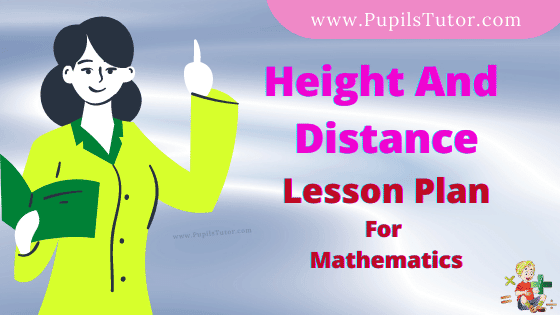Hello Friends, Welcome To Our Website. How Are You? Hope You Are Doing Well,

If You Are Searching For Height And Distance Lesson Plan Then You Have Come To The Right Place. Here We Have Shared The Lesson Plan On Height And Distance For B.Ed, D.El.Ed, And School Teachers.

This Simulated Teaching, Real School Teaching Math Lesson Plan On Distance And Height Is Specially Made For The B.Ed 1st And 2nd Year Students But All The Trainee Teacher And School Teachers Of All Classes Can Prepare Their Daily Teaching Plan Very Easily With The Help Of This Model Height And Distance Lesson Plan For Class 10 To 12.

Brief Overview Of The Lesson Plan

 Class 10th Topic Height And Distance Subject Math Lesson Plan Type Simulated Teaching, Real School Teaching Skill School Teaching

The Topics And Points That Are Covered In This Mathematic Lesson Plan On Height And Distance Are : Application Of Trigonometry In Finding Height And Distance, Angle Of Elevation, Angle Of Depression, Trigonometry And Trigonometric Functions, Trigonometric Ratio,  Height And Distance, Measure Height And Distance From Trigonometric Functions….....

## Height And Distance Lesson Plan –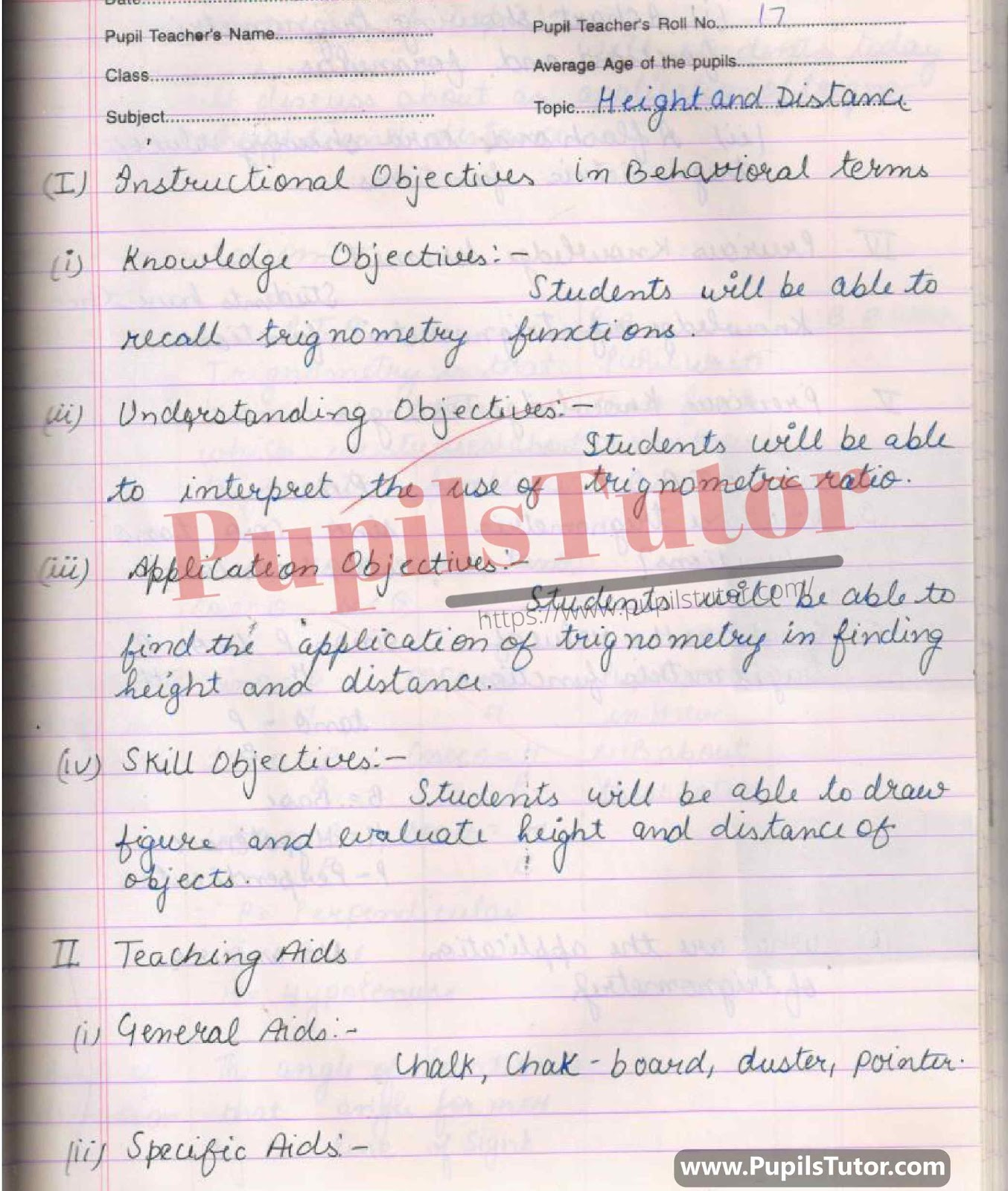## Mega And Real School Teaching Application Of Trigno, Height And Distance, Angle Of elevation In Trigno, Angle of Depression, Sine, Cosine, Cosecant, Secant, Cotangent, Tangent, Perpendicular(p), Base(b), Height(h) , Theta In Trigno Lesson Plan For B.Ed And Deled In English Free Download PDF And PPT –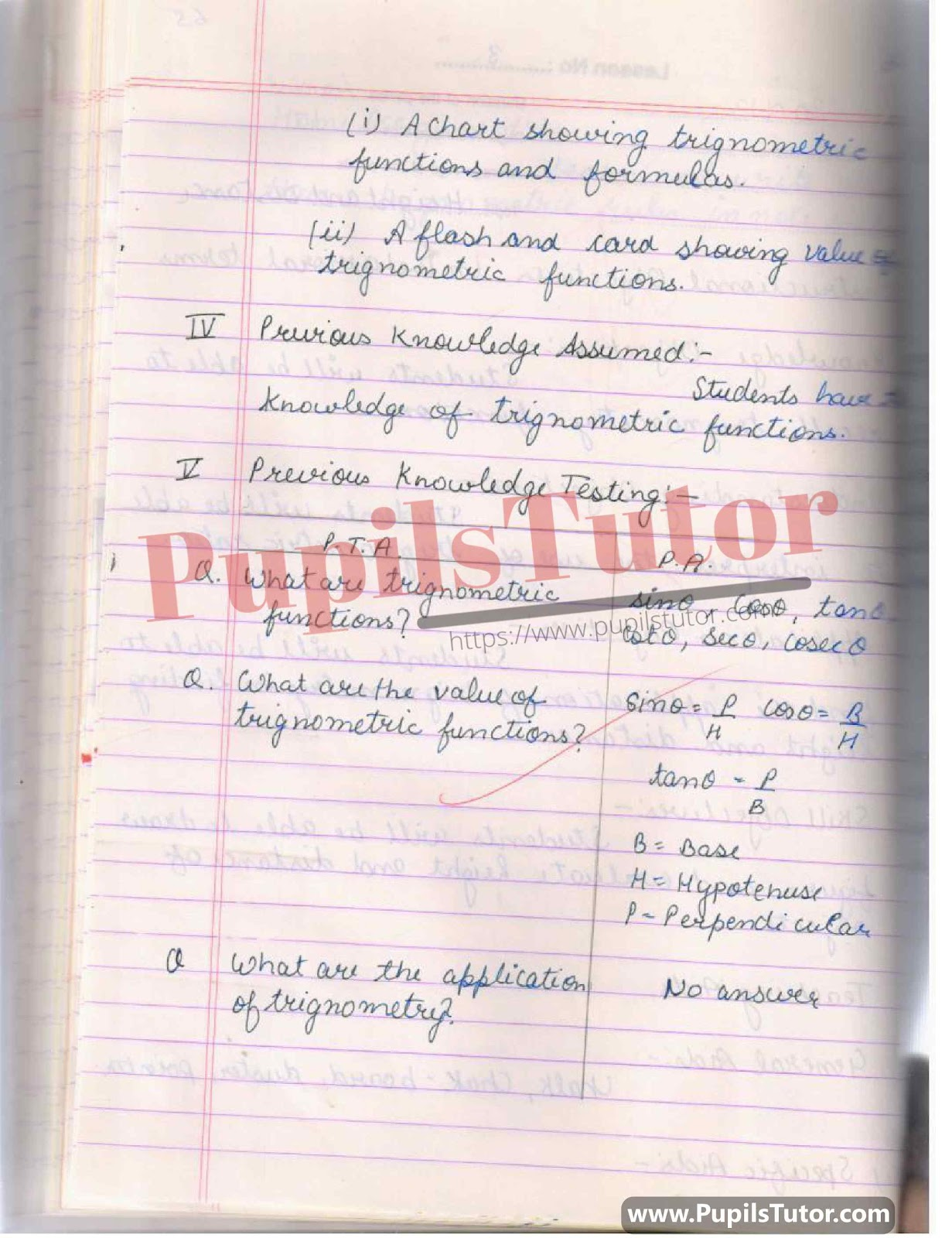## Math Lesson Plan On Trigonometry Application, Real Life Trigno Application, Height And Distance, Trigonometry Functions, Trigonometry Ratios, Trigonometry Identities, Sin, Cos, Tan, Cot, Sec, Cosec For Class/Grade 10 For CBSE NCERT School And College Teachers –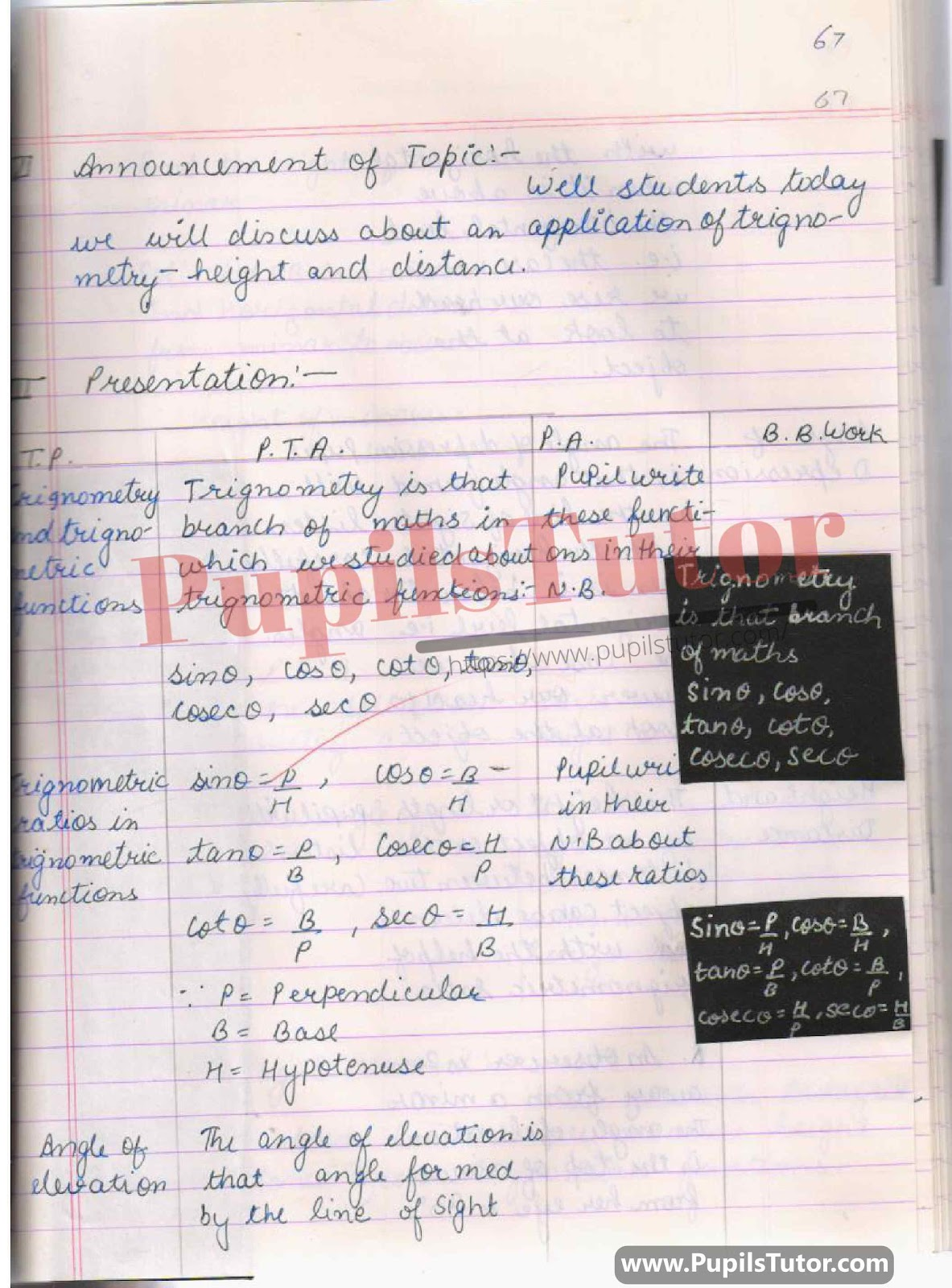## BED, DELED, BTC, BSTC, M.ED, DED And NIOS Teaching Of Mathematics Innovative Digital Lesson Plan Format On Angle Of Elevation, Angle Of Depression, Trigno, Trigno Identities, Trigno Ratio, Trigno Function, Height And Distance Topic For Class 9th, 10th, 11th, 12th –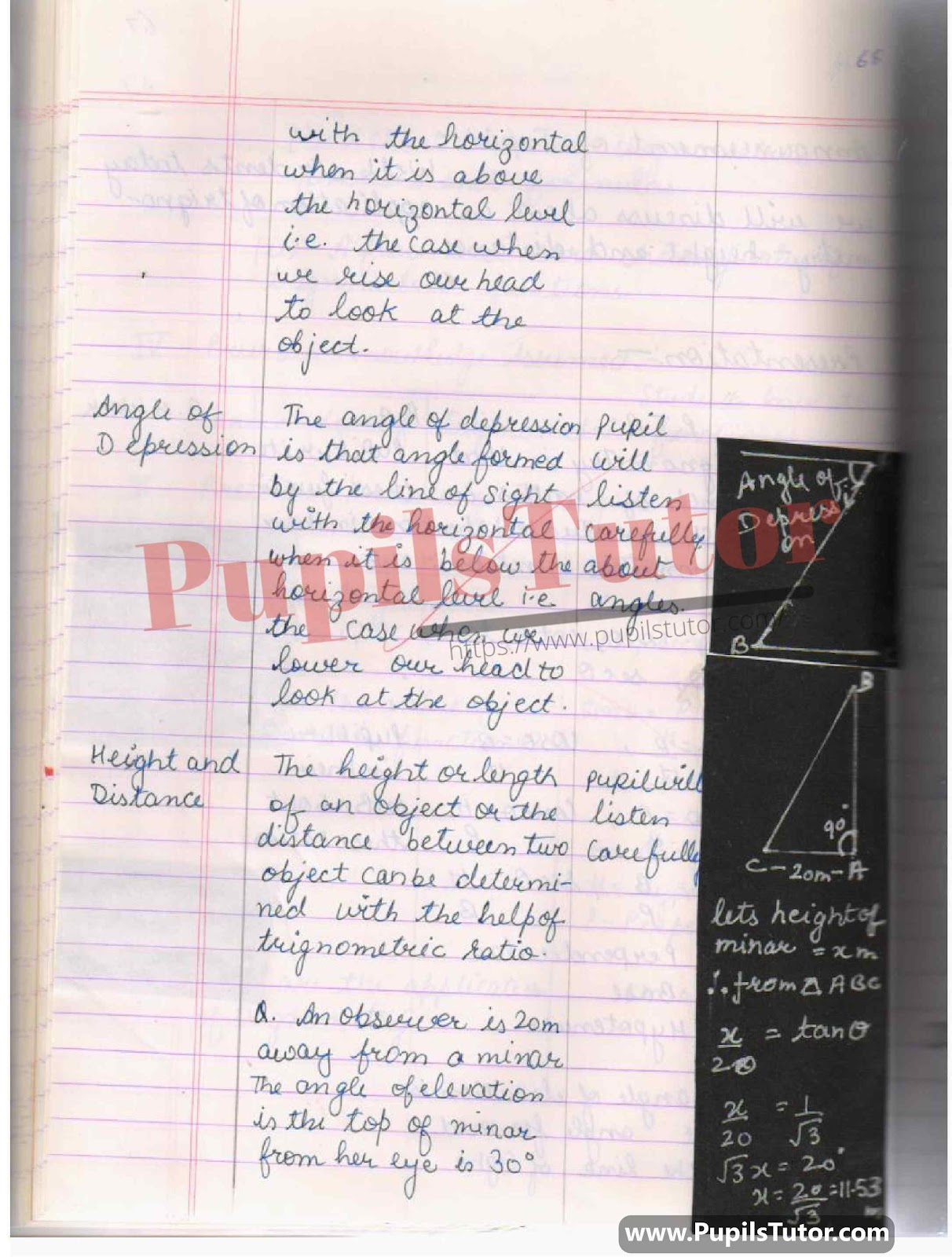## Best Lesson Plan On How To Measure Height And Distance From Trigonometry Functions with Examples And Homework, Questions-Answers Based on Trigonometry Application, Application Of Trigno In Finding Height And Distance of Building For Class 10. –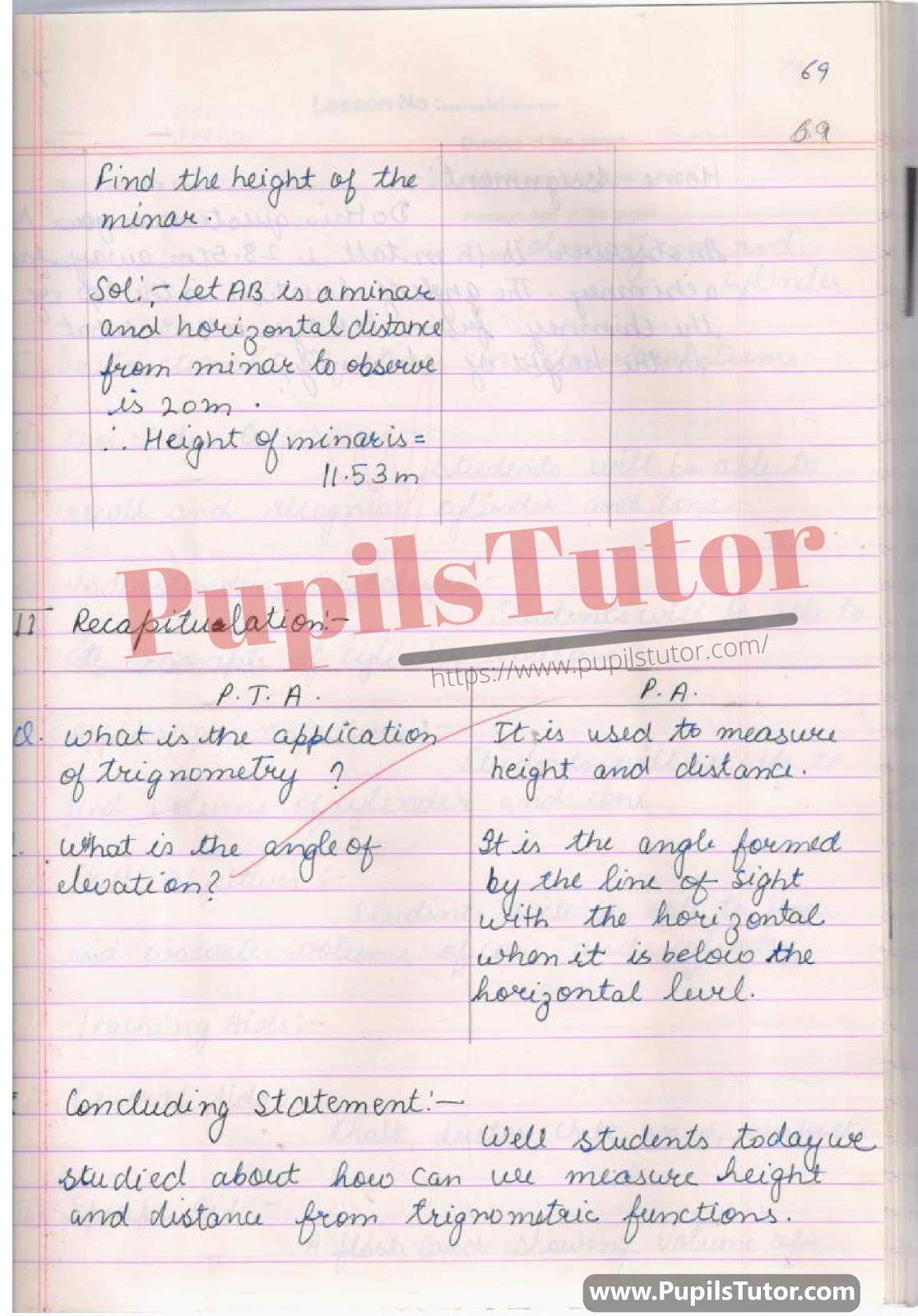## Define Angle Of Elevation, Define Angle Of Depression, Height And Distance, Trigno Application Simulated Teaching Skill Lesson Plan For B.Ed 1st Year, 2nd Year And All Semesters Students –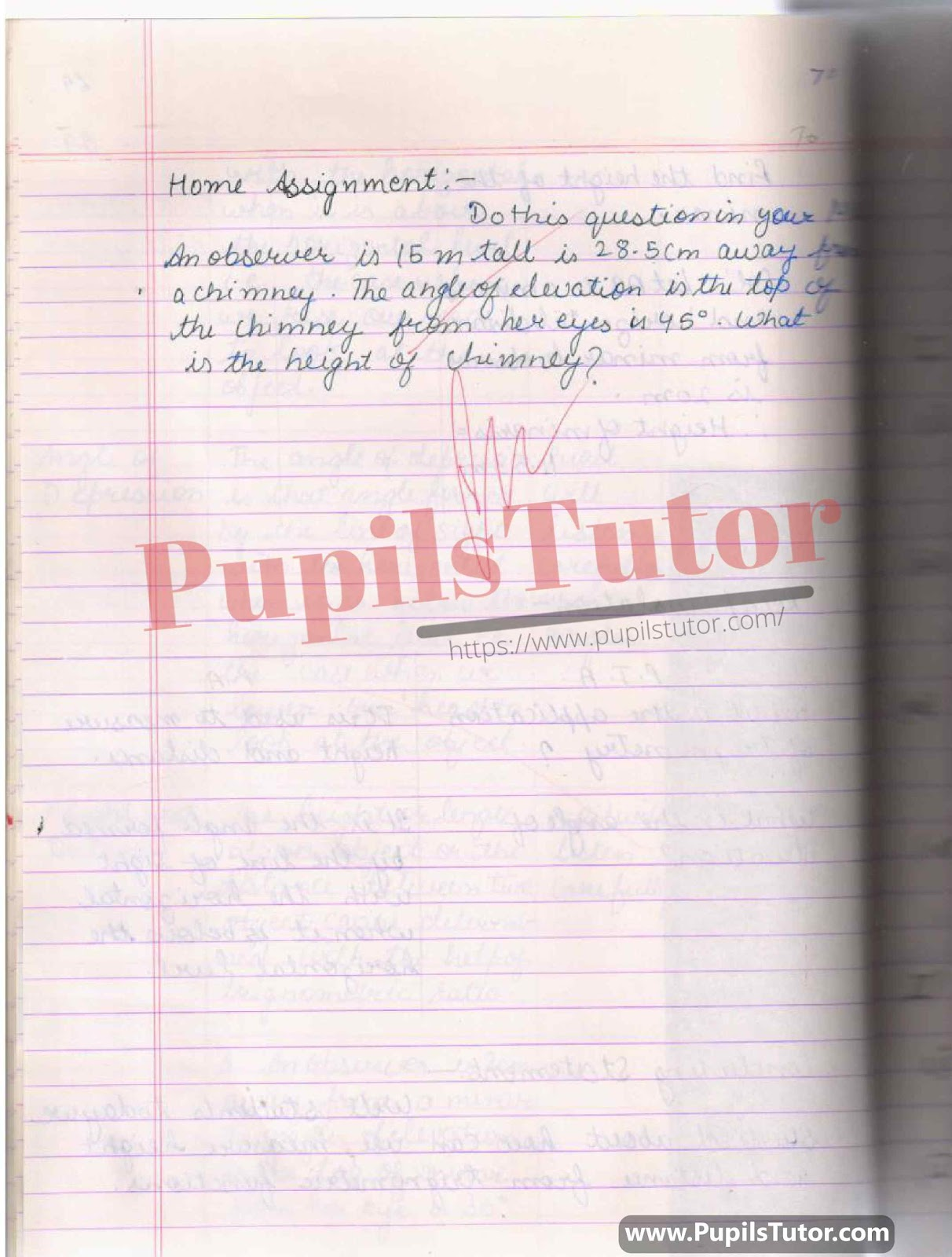You Might Also Like:

Further Reference

 B.Ed. Question Bank And Exam Notes A Complete Guide For B.ED. Entrance Examination CTET & TETs Preparation Material Teaching Aptitude and Attitude Test Book UP B.Ed Guidebook & Latest Practice Sets Book For Entrance Exam B.Ed CET Delhi University B.Ed Entrance Test Latest Practice Sets IGNOU B.Ed. Entrance Test: Previous Years Papers (Solved) Assignments And Files Lesson Planning Files And Guide

On Our Website www.PupilsTutor.Com, We Have Also Shared Lots Of Other Lesson Plans Of All Subjects On Various Teaching Skills Like Micro-Teaching, Mega Teaching, Discussion Skill, Real School Teaching And Practice Skill, Simulated And Observation Skills. You Can Also Check Them.

If You Liked This Height And Distance Lesson Plan For Class 10 And 11 Then Please Share Our Efforts With Your Friends Also.

You Can Also Share Your Lesson Plans, Assignments, Files, Papers And Study Notes With Us To Help Other Students .

#### List Of Some More Mathematics Lesson Plans

 Profit And Loss B.ed Maths Lesson Plan Sum Of The Angles Of A Triangle B.ed Maths Lesson Plan Trigonometric Ratio B.ed Maths Lesson Plan Circle B.ed Maths Lesson Plan Properties Of Angle In Circle B.ed Maths Lesson Plan Pie Chart B.ed Maths Lesson Plan 3 Dimensional Shape B.ed Maths Lesson Plan Trigonometry B.ed Maths Lesson Plan Parts Of A Circle B.ed Math Lesson Plan Compound Interest B.ed Math Lesson Plan Volume Of Cone And Cylinder Bed Maths Lesson Plan Areas Related To Circle B.ed Maths Lesson Plan Integer B.ed Maths Lesson Plan Surface Area Of Cone And Cylinder B.ed Maths Lesson Plan Statistics And Arithmetic Mean B.ed Maths Lesson Plan Volume Of Right Circular Cylinder B.ed Maths Lesson Plan Circular Trigonometry Function B.ed Maths Lesson Plan 3D Shapes B.ed Mathematics Lesson Plan Coordinate Geometry Section Formula B.ed Maths Lesson Plan Surface Area And Volume Of Sphere B.ed Maths Lesson Plan Theorem B.ed Maths Lesson Plan Curved And Total Surface Area Of Cylinder Bed Maths Lesson Plan Quadrilaterals B ed Maths Lesson Plan Height And Distance B.ed Maths Lesson Plan Curved And Total Surface Area Of Cone B.ed Maths Lesson Plan Coordinate Geometry B.ed Maths Lesson Plan Similar Triangle B.ed Maths Lesson Plan Polynomials B.ed Maths Lesson Plan Trigonometry Transformation Formula B.ed Maths Lesson Plan Surface Area Of Cube And Cuboid B.ed Math Lesson Plan Algebraic Expression B.ed Maths Lesson Plan Proportion B.ed Maths Lesson Plan